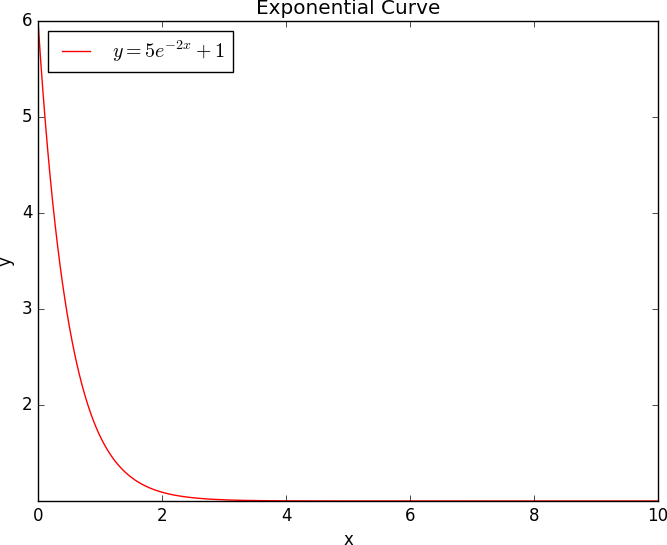### Exponential Graph using Python### Introduction to Exponential Graph

Exponential curve a is smooth and continues line of graph, connected by a series of co-ordinates calculated using a polynomial equation containing variable exponential value (For example, y = f(x), where f(x) = AeBx + C). In this program, I have used a polynomial equation with a exponential variable y = 5e-2x + 1 with x values range from 0 to 10. The program generated co-ordinate points (x, y) in the graph will be (0, 6.0), (1, 1.7), (2, 1.1), (3, 1.0), (4, 1.0), (5, 1.0), (6, 1.0), (7, 1.0), (8, 1.0), (9, 1.0), and (10, 1.0).

### Program Implementation

In this tutorial, I have used Python 3.5.2 (64-bit) software, and 7 modules: MatPlotLib 2.0.2, PyParsing 2.2.0, Python-DateUtil 2.6.1, PyTZ 2017.2, SetupTools 36.2.0, Cycler 0.10.0, and NumPy-MKL 1.13.1 implemented in Windows 10 Enterprise operating system. The 7 modules are chosen based on the compatibility of Python and OS version and bit.

#### Source Code

import numpy as np
import matplotlib.pyplot as plt

a = 5
b = 2
c = 1
x = np.linspace(0, 10, 256, endpoint = True)
y = (a * np.exp(-b*x)) + c

plt.plot(x, y, '-r', label=r'$y = 5e^{-2x} + 1$')

axes = plt.gca()
axes.set_xlim([x.min(), x.max()])
axes.set_ylim([y.min(), y.max()])

plt.xlabel('x')
plt.ylabel('y')
plt.title('Exponential Curve')
plt.legend(loc='upper left')

plt.show()